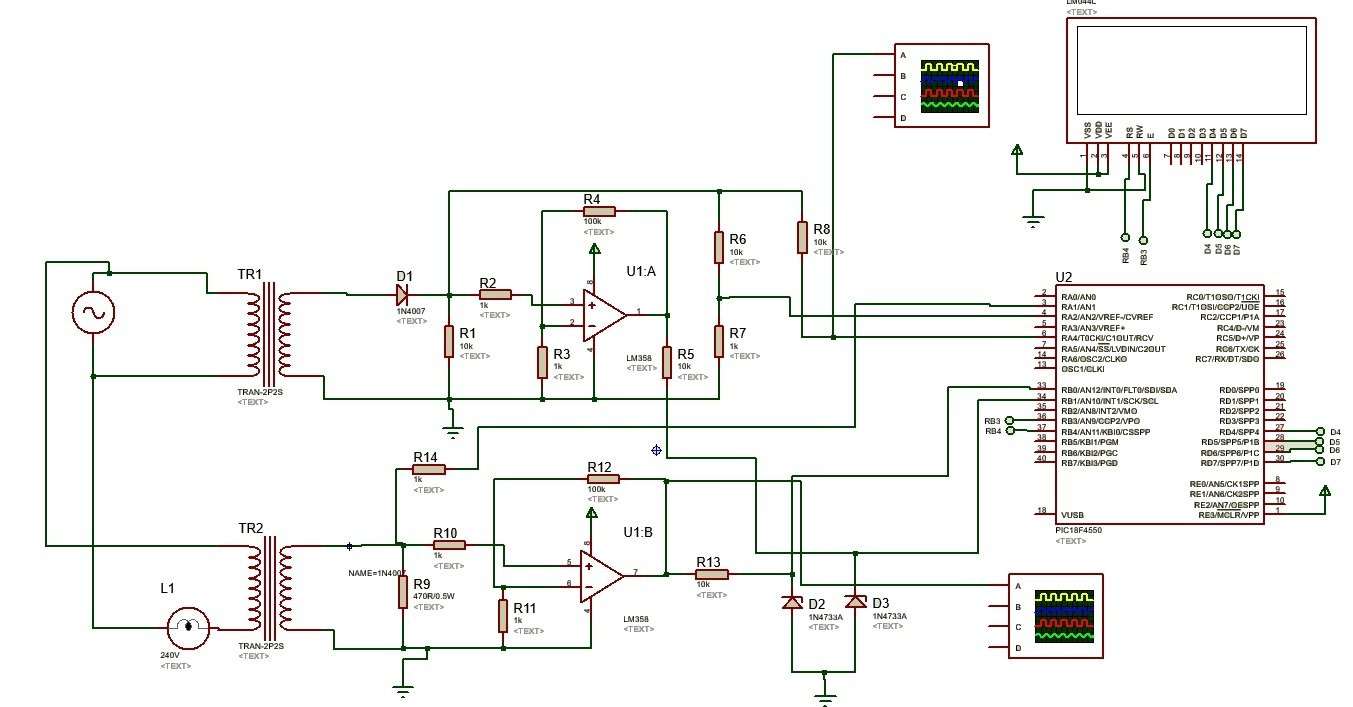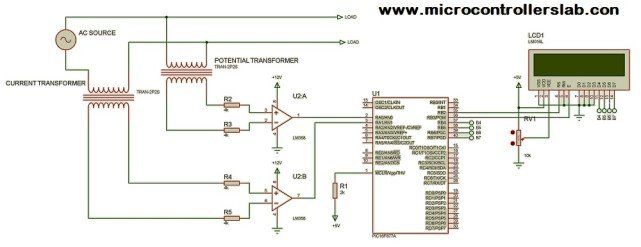# Digital watt meter using pic microcontroller

In this article, I will discuss how to design digital ac watt meter using pic microcontroller. How to measure ac power using pic microcontroller? Lets start with basic introduction of watt meter. Before you start with this article you should know basic understanding of pic microcontroller programming . if you want to learn pic microcontroller, check these pic microcontroller tutorials.

### What is Watt meter?

This pic microcontroller based project, Watt meter is electrical device which is used to measure real power in AC circuits and total power in DC circuits. You may have used watt meter in electrical labs. Watt meter most frequently used in electrical labs for performing various lab tasks.

#### How to measure AC power using pic microcontroller :

AC power is the sum of real power and reactive power. Watt meter is used to measure real power in electrical circuits. As you know real power or active power is the product of voltage, current and power factor of load. So to design AC watt meter using pic microcontroller, you should know how to measure AC voltage, AC current and Power factor using pic microcontroller. After measuring these parameters , one can easily design ac watt meter using pic microcontroller. Real power formula is given below:

P = V * I * Cos(θ)

Where P is real power. V is rms voltage across the load. I is rsm value of current across the load and Cosθ is power factor of load. So to measure active power with the help of pic microcontroller, one should measure above given parameters of real power using pic mcicrocontroller.

### How to measure AC current for digital watt meter:

AC current can be measured with the help of pic microcontroller using two methods. one is using difference amplifier and other is current transformer. I have already posted a article on how to measure ac current using pic microcontroller. you can check this article to know about how to measure ac current.

• AC current measurement using pic microcontroller  : In this project, I have used difference amplifier and shunt resistor to measure AC current. PIC16F877A microcontroller is used to measure AC current with the help of analog to digital converter. LCD display is used to display value of current on LCD.  Shunt resistor is used a transducers which converts current into voltage. Because microcontroller can not read current directly. Voltage across shunt resistor is measured with the help of pic microcontroller. This measured voltage is again converted back into current using ohm’s law formula. Because value of shunt resistor is known and voltage across shunt resistor is measured with the help of microcontroller. By using  V=IR foruma, current value can be easily calculated with some instructions of programming. I recommend you to read AC current measurement article before going further in this article.

### AC voltage measurement using pic microcontroller

AC voltage can also be measured easily using pic microcontroller. I have already posted two article on AC voltage measure using pic microcontroller. AC voltage measurement using pic microcontroller and Difference amplifier and AC voltage measurement using pic mcirocontroller and potential transformer.

### Power factor measurement using pic microcontroller

To measure power factor with the help of microcontroller, you should know about zero crossing detection using pic microcontroller. Power factor can be measured after measuring time between two consecutive zero crossing detection’s and time Difference between current and voltage wave form. To read more about zero crossing detection and power factor measurement using pic microcontroller, check following articles:

### Digital ac watt meter using pic microcontroller:

After reading after above articles, I hope that you know how to measure current, voltage and power factor using pic microcontroller. Now the question is how to design watt meter using these parameters. As you know watt meter is use to measure real power in AC circuits. Real power is product of voltage, current and power factor.

P = V * I * Cos(θ)

By using above formula you can calculate real power.  I have given all the details in above articles to measure, current and power factor.  You just need to multiply all these values in coding to measure real power.### Circuit diagram of digital watt meter using pic microcontroller:

Circuit diagram of digital watt meter using PIC16F877A microcontroller is given below. In this circuit diagram Current transformer and potential transformer is used to measure AC voltage and current. Difference amplifier is used for zero crossing detection.Liquid crystal display is used to display voltage, current, power factor and real power value. I have tried to give you all the information, you need to design digital watt meter using pic microcontroller. I recommend you to write your own code for digital watt meter. But if you want to hire us to write code for digital watt meter, contact us. Our team will provide you project service to write code for digital watt meter.

[button-brown url=”http://store.microcontrollerslab.com/product/digital-ac-watt-meter-using-pic-microcontroller/” target=”” position=”center”]Click here to purchase code and proteus simulation [/button-brown]

### 24 thoughts on “Digital watt meter using pic microcontroller”

1. where is the program ????????

• sir i have a project like implementation of ac wattmeter in micro-processor and microcontroller lab. Did this circuit is helpful for me.

can you provide us for the components used in this project .. what kind of transformer and is it available on amazon or ebay !!

if you had build such this circuit .. can you share picture if you don’t mind “ )

• you can contact me for project service as i have mentioned at the end of article

3. Hello
thanks a lot from your webpage.
I have a question?
(watt meter with pic)
In your circute:after the ct,We don’t need to rectifier before to connect lm385?
how we could ac signal to lm and then micro?
thanks for all
by

• there is no need to use rectifier in this circuit. All help is written in article read article.
if you need project service do let me know

4. Hi Mr Malik,

I am looking for a 3 phase ac voltage meter using arduino.

The sensor needs to be resistor network.

Pl guide me

5. Hi Bilal. I am mechatronical engineering student and i want to make a project about ac power measurement using pic microcontroller . So I need your project code . Please can you sen me your project code. This is my mail yurdakul5805@gmail.com

6. Hi , Mrs Bilal Malik, I am designing a project to verify calibration of electrical energy meters with a known Power of 200W applied as a function of voltage. It would be possible to do something like this with PIC.
thanks

7. please send a program for three phase ac power measurement by using pic18f4550

8. Hi I am interested in the single phase power mesurment but I also need to know if the power is being imported. Or exported could this be done by detecting the polarity of the voltage from the ct and comparing it with the polarity of the mains voltage signwave at the maximum voltage point of the mains ? I have just started to follow your page and it is helping me understand some of the applications for these devices. Many thanks. Bill

10. Ac power measurement using microC

11. I need source code (Digital watt meter using pic microcontroller) plz ,
this my email : hussam202@gmail.com
Thanks a lot
Regards

12. Hai,

We Interested purchase our source code of Digital Watt meter.

In our project, we require a differential amplifier circuit in order to measure the following measurements which includes 3 Phase AC voltage, current, power factor and phase angle of each phase along with a source code.

Then, we need a replacement of PIC Microcontroller with STM32F4 Discovery board.